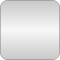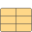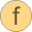# Common# Package ContentsPhaseBoundaryProperties Thermodynamic base properties on the phase boundaryInverseDerivatives_rhoT Derivatives required for inversion of density and temperature functions w.r.t. pressure and enthalpy statesEOSIdealCoeff Record for coefficients of ideal term of Helmholtz equation of stateEOSResidualCoeff Record for coefficients of residual term of Helmholtz equation of stateCubicSplineDerEval Derivative of cubic splineCubicSplineEval Cubic splinecv2Phase Compute isochoric specific heat capacity inside the two-phase regionFindInterval Half-interval search algorithmhelmholtzToBoundaryProps Calculate phase boundary property record from dimensionless Helmholtz function